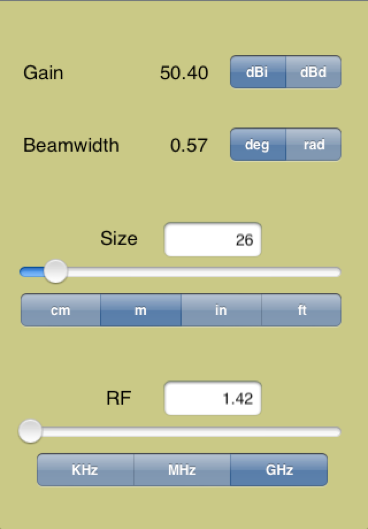Antenna Gain CalculatorAntenna Gain Calculator gives you a convenient way to compute the gain and beamwidth of parabolic reflectors based on the size and RF frequency. The calculator supports units of centimeters, meters, inches, and feet for size and KHz, MHz, and GHz for frequency.

The controls are two sliders which let you see the results of the computation - so if you need to achieve a certain gain at fixed RF it is very simple to adjust the size parameter and find the answer.

For high accuracy calculations you can use the keyboard to enter the values as well.

Calculate the Gain and BeamwidthSupport and info: a2s2@mac.com